Study Guide

# Basic Algebra - Slope

## Slope

The slope of a line measures its steepness; the larger the absolute value of the slope, the steeper the line is. If we don't know the slope, it's usually represented by the variable m. Why? Maybe because the French word for climb is "monter," but who knows? Some mathematician probably just chose m as the lucky letter.

Slope is calculated as change in the vertical direction (y) ÷ change in the horizontal direction (x). This is often called rise over run.### Calculating the Slope

One way to find the slope is to pick any two points, then count out how far we need to "rise" to get from the first point to the second point.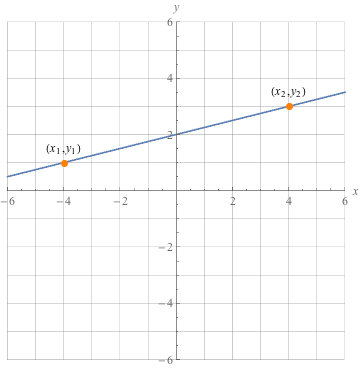In the graph above, we need to go up 2 spaces from the first point to be in line with the second point. This becomes our numerator.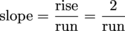Then we count how far we need to "run" horizontally to get from the first point to the second point. The second point is 8 units to the right of the first point. This becomes our denominator.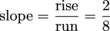We simplify the fraction and get a slope of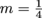.

We can also find the slope using a formula. Here's that formula.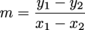We pick two points on the line and then we subtract the y-values to get the numerator. Then subtract the x-values and make this the denominator. The formula is super handy if the points are decimals or fractions. The important thing to remember is to keep the points in the same order in the numerator and denominator.In this line, we've picked the first point to be (-4, 1), so x1 = -4 and y1 = 1.

The second point is (4, 3), so x2 = 4 and y2 = 3.

Plugging these into the slope formula we get:So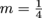, which is the same answer we got using the other method.

Also, we totally could've switched the coordinates and said that (x1, y1) = (4, 3) and (x2, y2) = (-4, 1) instead. Try it out:See? Same answer. We can start with either point, as long as we're careful to keep both x1 and y1 on the same side of the fraction.

Notice that if we could walk the line from the left to the right, we'd be walking uphill. This means that the line has a positive slope. Lo and behold, the slope is positive.

A positive slope tells us that the line goes uphill, from left to right. A negative slope tells us that the line goes downhill, from left to right.The two graphs on the left have steeper slopes, so the absolute value of their slopes is larger than the absolute value of the slopes on the right.

### 0 and Undefined Slopes

Let's look at two more examples of slope.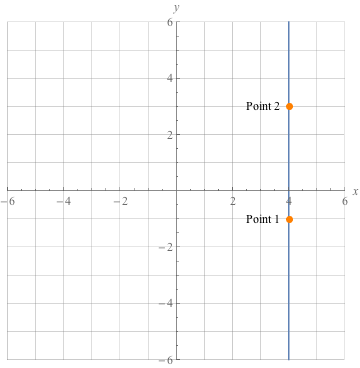To find the slope of this vertical line, we can start with either point, but let's pick (4, -1) as the first point and (4, 3) as the second point. Plug these into the slope equation.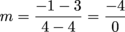Wait, something's wrong here—zeros aren't allowed in a denominator. What is the steepness of a vertical line? How can we measure the steepness of an infinitely steep line? We can't. To make our math brains feel better, we call this steepness undefined. All vertical lines have undefined slopes.

What about the slope of a horizontal line?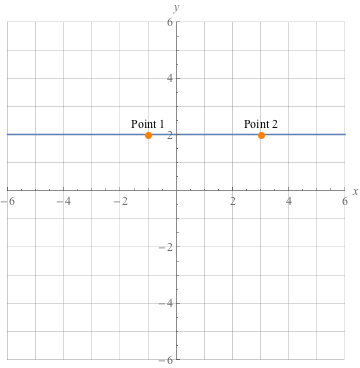Plop these points into the slope equation.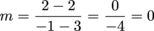We can have a zero as a numerator, which makes the value of the fraction 0. So the slope of a horizontal line is 0, which makes sense because it has no steepness at all.